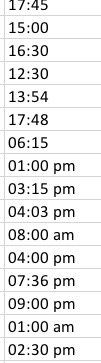# Multiple Time Formats in Import File

I need to import a column from Excel that contains times in two different formats per screenshot below.How can I make all times conform to the 24-hour format?

I will need to sort records based on this field. Since all time in Pan X is recorded in numbers, I have made the Time field an integer.

I have read other posts on Date type but I couldn’t find anything on Time.

Your friend in this case is the time( function:

Simplest answer: can’t you reformat in Excel to make what you’re importing consistent?

I have imported the XLS file into an Integer field. As expected, it ignored the am/pm.
Then I re-imported using a Text field and input the formula time(hh:mm) which is giving me an error a syntax error.
Where am I going wrong?

Yes, I can get this easily solved in Excel but I am trying to eliminate that step.

The time( function returns a number representing the time. The number is the number of seconds since midnight. If you have the XLS data in a text field you can have another integer field and use a formula to fill that field with time(myTextField) and it will convert the time as text to numeric values as time in seconds from midnight. You should not have to change the original XLS data at all since the time( function is smart enough to handle both AM/PM and 24 hour formats.

Rereading your original post I now see that you wanted to know how to turn that mixed list of variously formatted times into a common 24 hour format. Use this formula:

``````timepattern(time(«»),"hh:mm")
``````

The time( function will first convert the original text time into a numeric value and the timepattern( function will then reconvert this back to the 24 hour formatted text.

Since this is being done for sorting purposes, this needs to be modified a little, so that times before 10:00 will have a leading zero.

``````("0"+timepattern(time(«»),"hh:mm"))[-5,-1]
``````

The [-5,-1] text funnel at the end trims the string to the last 5 characters, which will trim off the leading zero when it is unneeded.

I have copied/pasted Dave’s formula and I get the error messageThere was probably a record where the time field was empty. Did it correctly fill those that had a time?

Gary’s formula would have been used in a formulafill in a text field that already had times that were inconsistently formatted. If this formula is being used in the import dialog, you would need to have the column number between the «» chevrons.

The first record had an empty time.
All working great now.
Much appreciated, all.